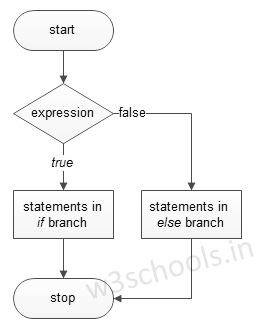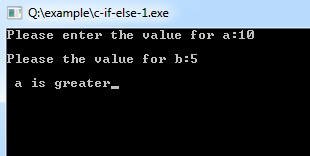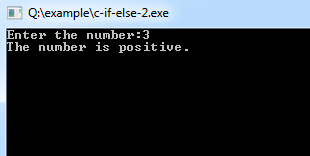##### C Programming Tutorial Index

C "If else statements" also control the program flow based on conditions such as "if statements"; the only difference is that it executes some statement code block if the expression is `true`; otherwise, it executes the else statement code block.

The basic format of if else Statement is:

Syntax:

``````if(test_expression)
{
}
else
{
}``````

Figure - Flowchart of if-else Statement:### Example of a C Program to Demonstrate if-else Statement

Example:

``````#include<stdio.h>

void main()
{
int a, b;

printf("Please enter the value for a:");
scanf("%d", &a);

scanf("%d", &b);

if (a > b) {
printf("\n a is greater");
} else {
printf("\n b is greater");
}
}``````

Program Output:Example:

``````#include<stdio.h>

void main() {
int num;
printf("Enter the number:");
scanf("%d", &num);

/* check whether the number is negative number */
if (num < 0)
printf("The number is negative.");
else
printf("The number is positive.");
}``````

Program Output:### if-else Statements in C - Video Tutorial

Please watch this video tutorial to understand "C if-else Statements" in more depth.